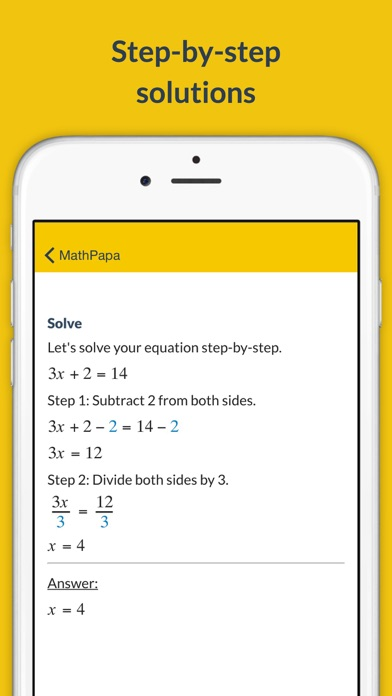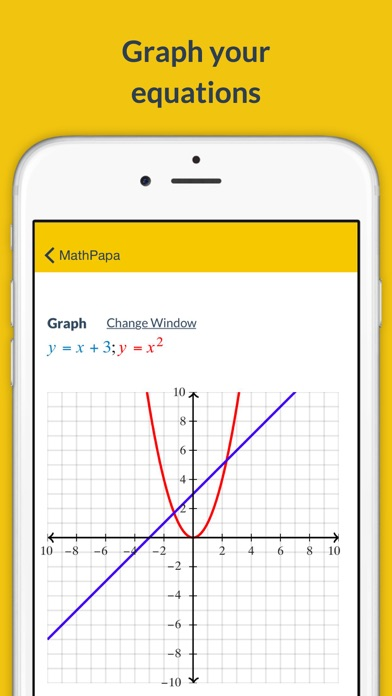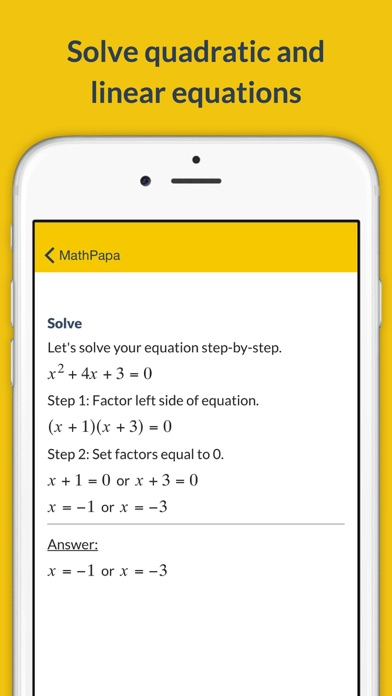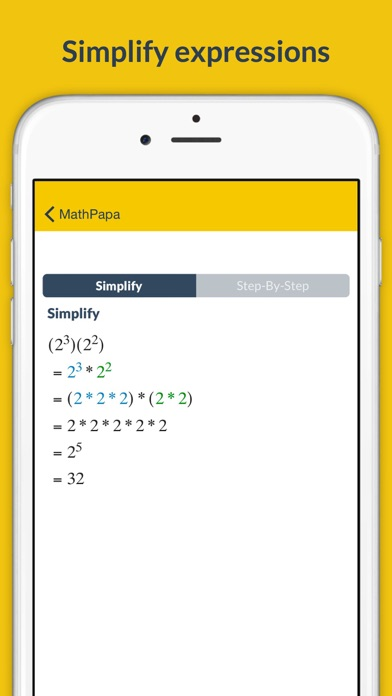# MathPapa - Algebra Calculator - Lumos Educational App Store4.712641
Price -Free
\$0

#### DESCRIPTION:

SOLVE your algebra problems step-by-step with MathPapa! MathPapa can solve your equations (and show the work!) and help you when you're stuck on your math homework. FEATURES: • Solves linear equations and quadratic equations. • Solves linear and quadratic inequalities. • Graphs equations. • Factors quadratic expressions. • Order of operations step-by-step. • Evaluates expressions. • Solves systems of two equations. STEP-BY-STEP SOLUTIONS: MathPapa's goal is to help you learn algebra step-by-step. Get help on your algebra problems with the MathPapa Algebra Calculator! HOW TO

#### OVERVIEW:

MathPapa - Algebra Calculator is a free educational mobile app By MathPapa, Inc..It helps students practice the following standards .

This page not only allows students and teachers download MathPapa - Algebra Calculator but also find engaging Sample Questions, Videos, Pins, Worksheets, Books related to the following topics.

#### ADDITIONAL INFORMATION:

Developer: MathPapa, Inc.

Developer URL: http://www.mathpapa.com/

Software Version: 1.4.2

Category: Education

Release Date: 2015-08-31T17:56:42Z#### Are you the Developer?EdSearch WebSearch